Balbharti 12th Maharashtra State Board Maths Solutions Book Pdf Chapter 6 Differential Equations Ex 6.3 Questions and Answers.

## Maharashtra State Board 12th Maths Solutions Chapter 6 Differential Equations Ex 6.3

Question 1.
In each of the following examples verify that the given expression is a solution of the corresponding differential equation.
(i) xy = log y + c; $$\frac{d y}{d x}=\frac{y^{2}}{1-x y}$$
Solution:
xy = log y + c
Differentiating w.r.t. x, we get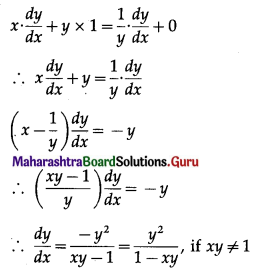Hence, xy = log y + c is a solution of the D.E.
$$\frac{d y}{d x}=\frac{y^{2}}{1-x y^{\prime}}, x y \neq 1$$

(ii) y = (sin-1x)2 + c; (1 – x2) $$\frac{d^{2} y}{d x^{2}}-x \frac{d y}{d x}=2$$
Solution:
y = (sin-1 x)2 + c …….(1)
Differentiating w.r.t. x, we get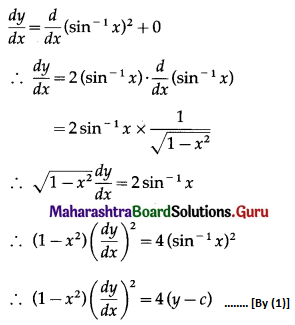Differentiating again w.r.t. x, we get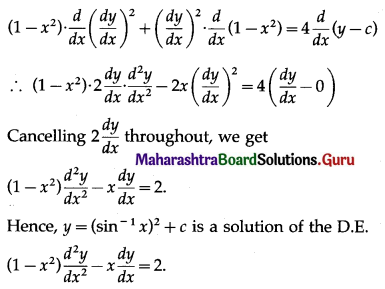(iii) y = e-x + Ax + B; $$e^{x} \frac{d^{2} y}{d x^{2}}=1$$
Solution:
y = e-x + Ax + B
Differentiating w.r.t. x, we get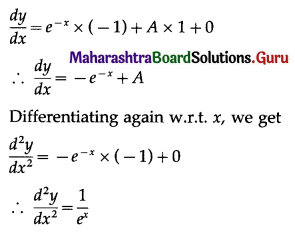∴ $$e^{x} \frac{d^{2} y}{d x^{2}}=1$$
Hence, y = e-x + Ax + B is a solution of the D.E.
$$e^{x} \frac{d^{2} y}{d x^{2}}=1$$

(iv) y = xm; $$x^{2} \frac{d^{2} y}{d x^{2}}-m x \frac{d y}{d x}+m y=0$$
Solution:
y = xm
Differentiating twice w.r.t. x, we get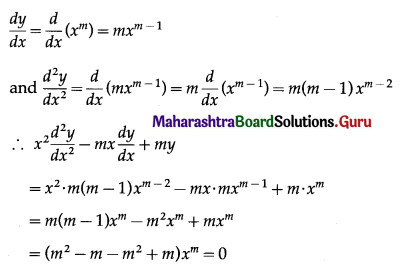This shows that y = xm is a solution of the D.E.
$$x^{2} \frac{d^{2} y}{d x^{2}}-m x \frac{d y}{d x}+m y=0$$

(v) y = a + $$\frac{b}{x}$$; $$x \frac{d^{2} y}{d x^{2}}+2 \frac{d y}{d x}=0$$
Solution:
y = a + $$\frac{b}{x}$$
Differentiating w.r.t. x, we get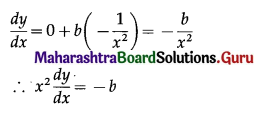Differentiating again w.r.t. x, we get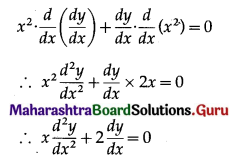Hence, y = a + $$\frac{b}{x}$$ is a solution of the D.E.
$$x \frac{d^{2} y}{d x^{2}}+2 \frac{d y}{d x}=0$$(vi) y = eax; x $$\frac{d y}{d x}$$ = y log y
Solution:
y = eax
log y = log eax = ax log e
log y = ax …….(1) ……..[∵ log e = 1]
Differentiating w.r.t. x, we get
$$\frac{1}{y} \cdot \frac{d y}{d x}$$ = a × 1
∴ $$\frac{d y}{d x}$$ = ay
∴ x $$\frac{d y}{d x}$$ = (ax)y
∴ x $$\frac{d y}{d x}$$ = y log y ………[By (1)]
Hence, y = eax is a solution of the D.E.
x $$\frac{d y}{d x}$$ = y log y.

Question 2.
Solve the following differential equations.
(i) $$\frac{d y}{d x}=\frac{1+y^{2}}{1+x^{2}}$$
Solution: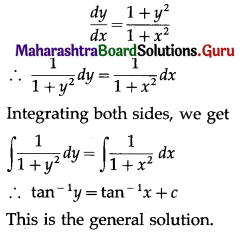(ii) log($$\frac{d y}{d x}$$) = 2x + 3y
Solution: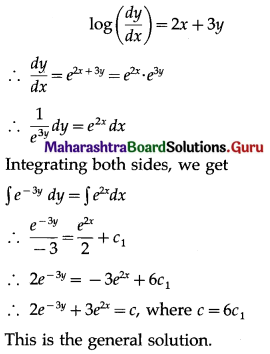(iii) y – x $$\frac{d y}{d x}$$ = 0
Solution:
y – x $$\frac{d y}{d x}$$ = 0
∴ x $$\frac{d y}{d x}$$ = y
∴ $$\frac{1}{x} d x=\frac{1}{y} d y$$
Integrating both sides, we get
$$\int \frac{1}{x} d x=\int \frac{1}{y} d y$$
∴ log |x| = log |y| + log c
∴ log |x| = log |cy|
∴ x = cy
This is the general solution.(iv) sec2x . tan y dx + sec2y . tan x dy = 0
Solution:
sec2x . tan y dx + sec2y . tan x dy = 0
∴ $$\frac{\sec ^{2} x}{\tan x} d x+\frac{\sec ^{2} y}{\tan y} d y=0$$
Integrating both sides, we get
$$\int \frac{\sec ^{2} x}{\tan x} d x+\int \frac{\sec ^{2} y}{\tan y} d y=c_{1}$$
Each of these integrals is of the type
$$\int \frac{f^{\prime}(x)}{f(x)} d x$$ = log |f(x)| + c
∴ the general solution is
∴ log|tan x| + log|tan y | = log c, where c1 = log c
∴ log |tan x . tan y| = log c
∴ tan x . tan y = c
This is the general solution.

(v) cos x . cos y dy – sin x . sin y dx = 0
Solution:
cos x . cos y dy – sin x . sin y dx = 0
$$\frac{\cos y}{\sin y} d y-\frac{\sin x}{\cos x} d x=0$$
Integrating both sides, we get
∫cot y dy – ∫tan x dx = c1
∴ log|sin y| – [-log|cos x|] = log c, where c1 = log c
∴ log |sin y| + log|cos x| = log c
∴ log|sin y . cos x| = log c
∴ sin y . cos x = c
This is the general solution.

(vi) $$\frac{d y}{d x}$$ = -k, where k is a constant.
Solution:
$$\frac{d y}{d x}$$ = -k
∴ dy = -k dx
Integrating both sides, we get
∫dy = -k∫dx
∴ y = -kx + c
This is the general solution.(vii) $$\frac{\cos ^{2} y}{x} d y+\frac{\cos ^{2} x}{y} d x=0$$
Solution:
$$\frac{\cos ^{2} y}{x} d y+\frac{\cos ^{2} x}{y} d x=0$$
∴ y cos2y dy + x cos2x dx = 0
∴ $$x\left(\frac{1+\cos 2 x}{2}\right) d x+y\left(1+\frac{\cos 2 y}{2}\right) d y=0$$
∴ x(1 + cos 2x) dx + y(1 + cos 2y) dy = 0
∴ x dx + x cos 2x dx + y dy+ y cos 2y dy = 0
Integrating both sides, we get
∫x dx + ∫y dy + ∫x cos 2x dx + ∫y cos 2y dy = c1 ……..(1)
Using integration by parts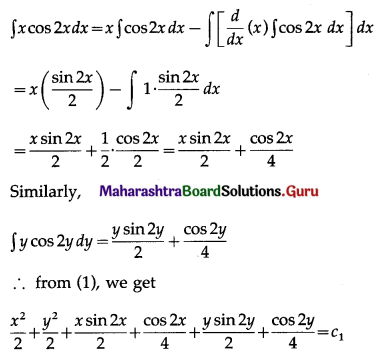Multiplying throughout by 4, this becomes
2x2 + 2y2 + 2x sin 2x + cos 2x + 2y sin 2y + cos 2y = 4c1
∴ 2(x2 + y2) + 2(x sin 2x + y sin 2y) + cos 2y + cos 2x + c = 0, where c = -4c1
This is the general solution.

(viii) $$y^{3}-\frac{d y}{d x}=x^{2} \frac{d y}{d x}$$
Solution: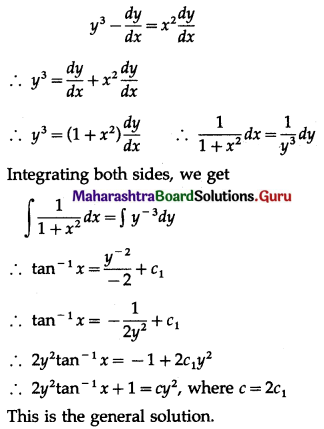(ix) 2ex+2y dx – 3 dy = 0
Solution: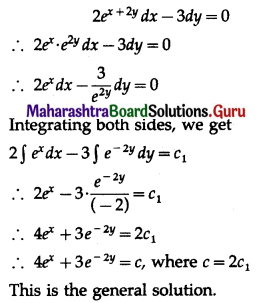(x) $$\frac{d y}{d x}$$ = ex+y + x2 ey
Solution: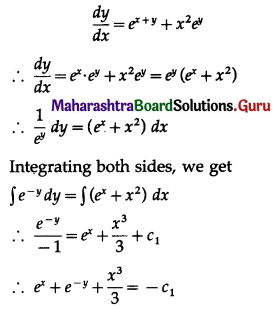∴ 3ex + 3e-y + x3 = -3c1
∴ 3ex + 3e-y + x3 = c, where c = -3c1
This is the general solution.

Question 3.
For each of the following differential equations, find the particular solution satisfying the given condition:
(i) 3ex tan y dx + (1 + ex) sec2y dy = 0, when x = 0, y = π
Solution:
3ex tan y dx + (1 + ex) sec2y dy = 0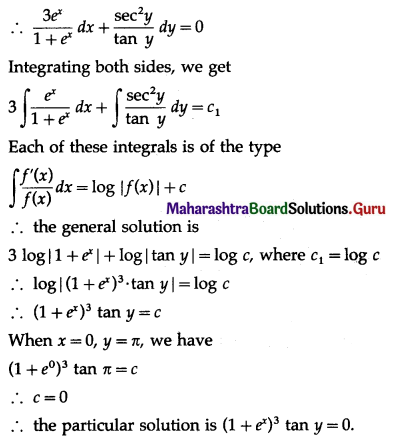(ii) (x – y2x) dx – (y + x2y) dy = 0, when x = 2, y = 0
Solution:
(x – y2x) dx – (y + x2y) dy = 0
∴ x(1 – y2) dx – y(1 + x2) dy = 0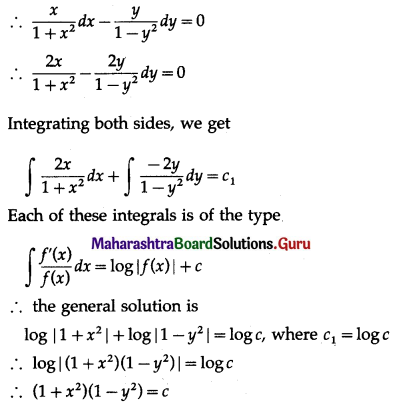When x = 2, y = 0, we have
(1 + 4)(1 – 0) = c
∴ c = 5
∴ the particular solution is (1 + x2)(1 – y2) = 5.

(iii) y(1 + log x) $$\frac{d x}{d y}$$ – x log x = 0, y = e2, when x = e
Solution:
y(1 + log x) $$\frac{d x}{d y}$$ – x log x = 0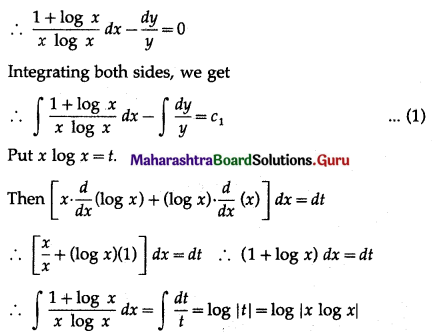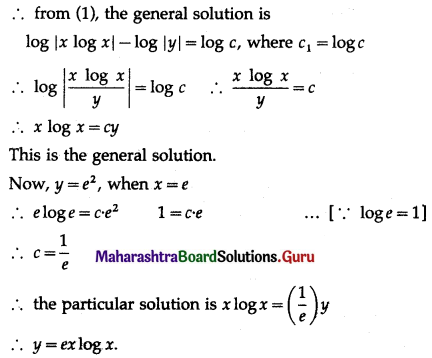(iv) (ey + 1) cos x + ey sin x $$\frac{d y}{d x}$$ = 0, when x = $$\frac{\pi}{6}$$, y = 0
Solution:
(ey + 1) cos x + ey sin x $$\frac{d y}{d x}$$ = 0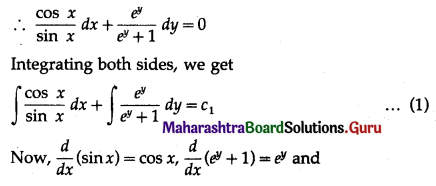$$\int \frac{f^{\prime}(x)}{f(x)} d x$$ = log|f(x)| + c
∴ from (1), the general solution is
log|sin x| + log|ey + 1| = log c, where c1 = log c
∴ log|sin x . (ey + 1)| = log c
∴ sin x . (ey + 1) = c
When x = $$\frac{\pi}{4}$$, y = 0, we get
$$\left(\sin \frac{\pi}{4}\right)\left(e^{0}+1\right)=c$$
∴ c = $$\frac{1}{\sqrt{2}}$$(1 + 1) = √2
∴ the particular solution is sin x . (ey + 1) = √2

(v) (x + 1) $$\frac{d y}{d x}$$ – 1 = 2e-y, y = 0, when x = 1
Solution: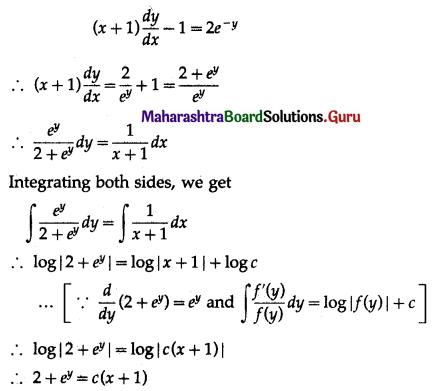This is the general solution.
Now, y = 0, when x = 1
∴ 2 + e0 = c(1 + 1)
∴ 3 = 2c
∴ c = $$\frac{3}{2}$$
∴ the particular solution is 2 + ey = $$\frac{3}{2}$$ (x + 1)
∴ 2(2 + ey) = 3(x + 1).(vi) cos($$\frac{d y}{d x}$$) = a, a ∈ R, y (0) = 2
Solution:
cos($$\frac{d y}{d x}$$) = a
∴ $$\frac{d y}{d x}$$ = cos-1 a
∴ dy = (cos-1 a) dx
Integrating both sides, we get
∫dy = (cos-1 a) ∫dx
∴ y = (cos-1 a) x + c
∴ y = x cos-1 a + c
This is the general solution.
Now, y(0) = 2, i.e. y = 2,
when x = 0, 2 = 0 + c
∴ c = 2
∴ the particular solution is
∴ y = x cos-1 a + 2
∴ y – 2 = x cos-1 a
∴ $$\frac{y-2}{x}$$ = cos-1a
∴ cos($$\frac{y-2}{x}$$) = a

Question 4.
Reduce each of the following differential equations to the variable separable form and hence solve:
(i) $$\frac{d y}{d x}$$ = cos(x + y)
Solution: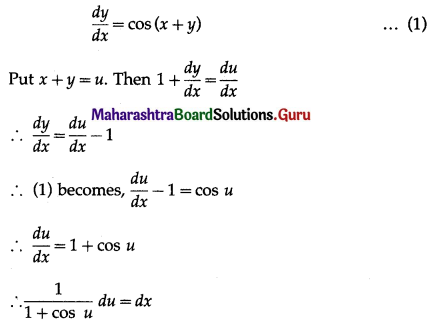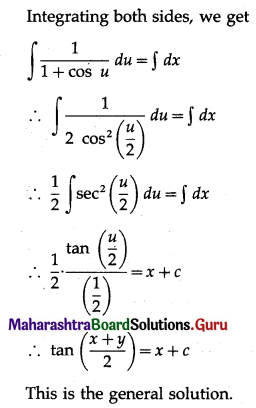(ii) (x – y)2 $$\frac{d y}{d x}$$ = a2
Solution: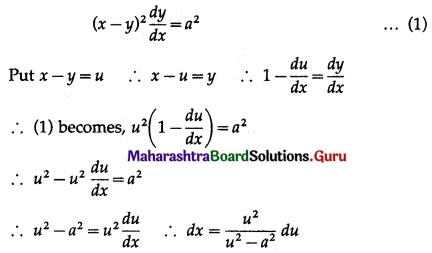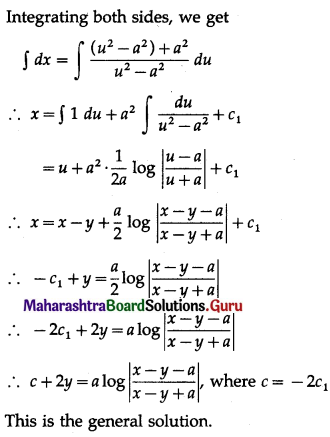(iii) x + y $$\frac{d y}{d x}$$ = sec(x2 + y2)
Solution: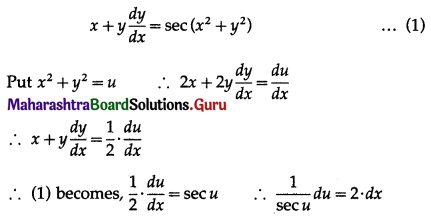Integrating both sides, we get
∫cos u du = 2 ∫dx
∴ sin u = 2x + c
∴ sin(x2 + y2) = 2x + c
This is the general solution.

(iv) cos2(x – 2y) = 1 – 2 $$\frac{d y}{d x}$$
Solution: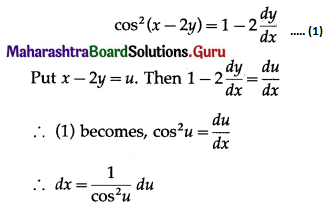Integrating both sides, we get
∫dx = ∫sec2u du
∴ x = tan u + c
∴ x = tan(x – 2y) + c
This is the general solution.(v) (2x – 2y + 3) dx – (x – y + 1) dy = 0, when x = 0, y = 1
Solution:
(2x – 2y + 3) dx – (x – y + 1) dy = 0
∴ (x – y + 1) dy = (2x – 2y + 3) dx
∴ $$\frac{d y}{d x}=\frac{2(x-y)+3}{(x-y)+1}$$ ………(1)
Put x – y = u, Then $$1-\frac{d y}{d x}=\frac{d u}{d x}$$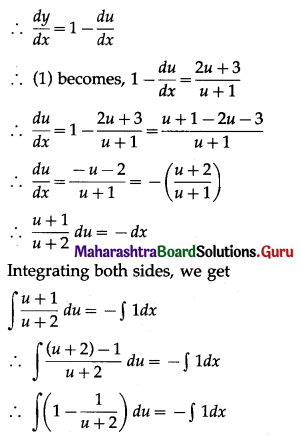∴ u – log|u + 2| = -x + c
∴ x – y – log|x – y + 2| = -x + c
∴ (2x – y) – log|x – y + 2| = c
This is the general solution.
Now, y = 1, when x = 0.
∴ (0 – 1) – log|0 – 1 + 2| = c
∴ -1 – o = c
∴ c = -1
∴ the particular solution is
(2x – y) – log|x – y + 2| = -1
∴ (2x – y) – log|x – y + 2| + 1 = 0